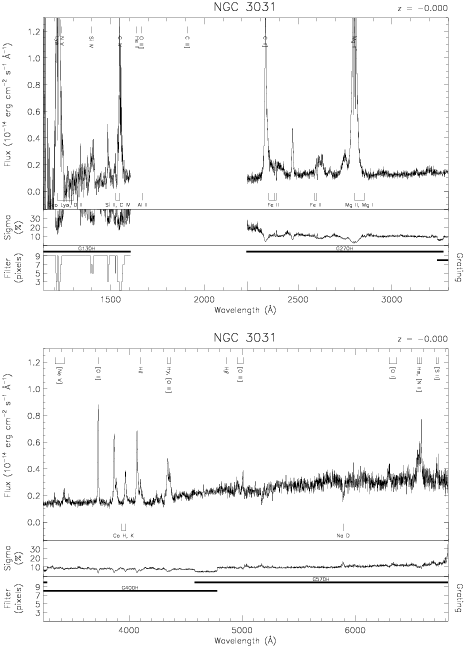Download dataset
TELESCOP= 'HST     '
INSTRUME= 'FOS     '
RADECSYS= 'FK5     '
EQUINOX =               2000.0
DATE-OBS= '1993-04-05T16:06:47'
RA_PNT  =       148.8890679167
DEC_PNT =        69.0653225000
PA_PNT  =       255.4480513941
OBJECT  = 'NGC 3031'
ROOTNM01= 'Y1AU0103T'
GRNDMD01= 'SPECTROSCOPY'
DETECT01= 'AMBER   '
APERID01= 'B-2     '
FGWAID01= 'H57     '
ROOTNM02= 'Y1AU0104T'
GRNDMD02= 'SPECTROSCOPY'
DETECT02= 'AMBER   '
APERID02= 'B-2     '
FGWAID02= 'H40     '
ROOTNM03= 'Y1AU0105T'
GRNDMD03= 'SPECTROSCOPY'
DETECT03= 'AMBER   '
APERID03= 'B-2     '
FGWAID03= 'H27     '
ROOTNM04= 'Y1AU0204T'
GRNDMD04= 'SPECTROSCOPY'
DETECT04= 'BLUE    '
APERID04= 'B-2     '
FGWAID04= 'H13     '
ROOTNM05= 'Y1AU0205T'
GRNDMD05= 'SPECTROSCOPY'
DETECT05= 'BLUE    '
APERID05= 'B-2     '
FGWAID05= 'H13     '